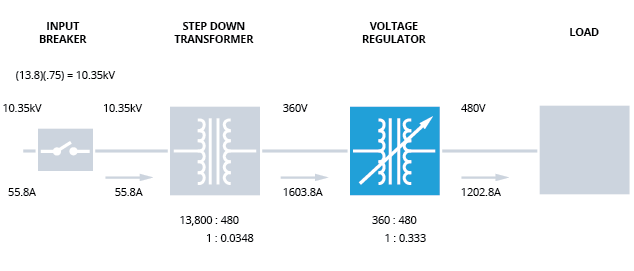#### 2.3.2 CASE 2: SYSTEM VOLTAGE 25% LOWFIGURE 4: Input voltage 25% low. Regulator boosts input voltage from 360V to 480V.

Here we assume that the input voltage is 25% low. For a nominal 13.8kV system supplying a 1000 kVA load, this means that input voltage is 10.35kV with a line current of 55.8 amperes. In this case, the line current is essentially the same as the input breaker rating – a less-than-desirable situation. Since the step-down transformer has a fixed transformation ratio, the low-side voltage will be 360 volts, and the current passing through the low voltage winding will be 1603.8 amperes.

The voltage regulator will address this situation by adjusting its turns ratio to boost the voltage by 33% (a turns ratio of 0.75 to 1.00). For the regulator to adequately address the low-voltage input, the regulator’s input winding and the step-down transformer’s output winding must be capable of carrying 1603.8 amperes. In other words, the step-down transformer’s secondary winding must be capable of conducting 33% more current than its nominal design capability over the duration of the voltage sag.

Next: 3. Conclusion